Open in App
Not now

# Flutter and Blockchain – Population Dapp

• Last Updated : 11 May, 2022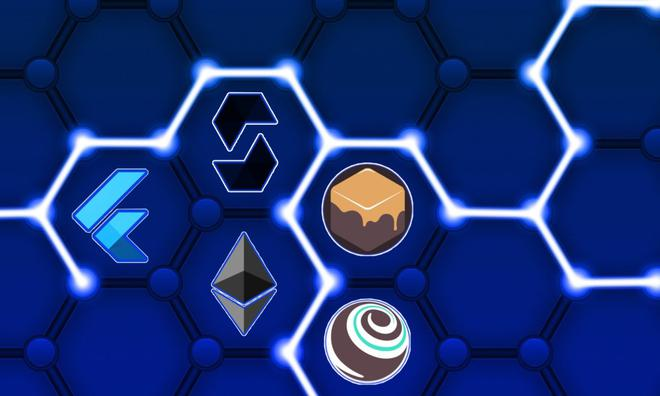Before checking out this article, Do take a look at Flutter and Blockchain – Hello World Dapp. This tutorial will take you through the process of building your mobile dapp – Population on Blockchain!

This tutorial is meant for those with a basic knowledge of Ethereum and smart contracts, who have some knowledge of the Flutter framework but are new to mobile dapps.

In this tutorial we will be covering:

1. Setting up the development environment
2. Creating a Truffle Project
3. Writing the Smart Contract
4. Compiling and Migrating the Smart Contract
5. Testing the Smart Contract
7. Creating a UI to interact with the smart contract
8. Interacting with the complete Dapp

### Description

Population on blockchain is a simple decentralized application, which will allow you to store a specific country population on the blockchain, you can increment as well as decrement the population based on the current condition of the country.

Output: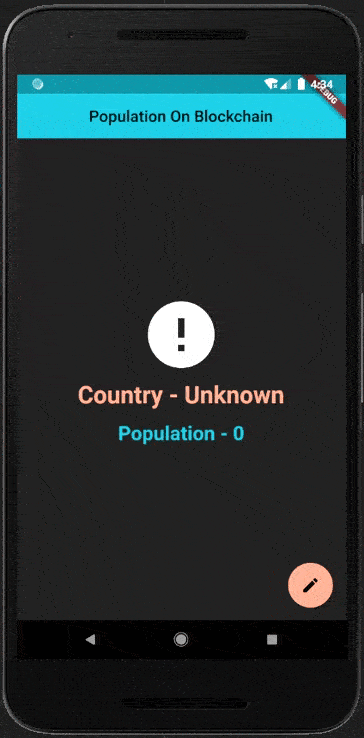## Setting up the development environment

Truffle is the most popular development framework for Ethereum with a mission to make your life a whole lot easier. But before we install truffle make sure to install node .

Once we have node installed, we only need one command to install Truffle:

`npm install -g truffle`

We will also be using Ganache, a personal blockchain for Ethereum development you can use to deploy smart contracts, develop applications, and run tests. You can download Ganache by navigating to https://truffleframework.com/ganache and clicking the “Download” button.

## Creating a Truffle Project

1. Create a basic Flutter project in your favorite IDE
2. Initialize Truffle in the flutter project directory by running
`truffle init`

### Directory Structure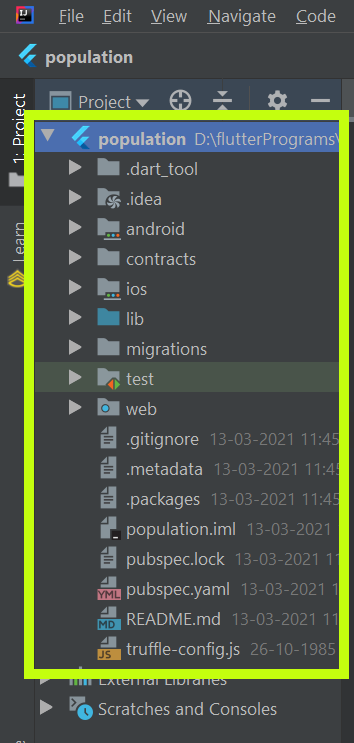• contracts/ : Contains solidity contract file.
• migrations/ : Contains migration script files (Truffle uses a migration system to handle contract deployment).
• test/ : Contains test script files.
• truffle-config.js : Contains truffle deployment configurations information.

## Writing the Smart Contract

The Smart Contract actually acts as the back-end logic and storage for our Dapp.

• Create a new file named Population.sol in the contracts/ directory.
• Add the following content to the file:

## Solidity

 `pragma solidity ^0.5.0; ` ` `  `contract` `Population { ` `     `  `} `

• The minimum version of Solidity required is noted at the top of the contract: pragma solidity ^0.5.9;.
• Statements are terminated with semicolons.

### Variable setup

• Add the following variable on the next line after contract Population {

## Solidity

 `string ``public` `countryName ; ` `uint ``public` `currentPopulation ;`

• countryName and currentPopulation will hold the name and population of the country.
• It is defined as public modifier because solidity automatically creates getter functions for all public state variables.

## Solidity

 `constructor() ``public``{ ` `  ``countryName = ``"Unknown"` `; ` `  ``currentPopulation = 0 ; ` `}`

Constructor in solidity is executed only once, when a contract is created and it is used to initialize contract state. Here we’re just setting the initial value of the variables.

### Function

1. Add the following function to the smart contract after the constructor declaration we set up above.

## Solidity

 `function set(string memory name, uint popCount) ``public``{ ` `  ``countryName = name ; ` `  ``currentPopulation = popCount ; ` `} ` ` `  `function decrement(uint decrementBy) ``public``{ ` `  ``currentPopulation -= decrementBy ; ` `} ` ` `  ` `  `function increment(uint incrementBy) ``public``{ ` `  ``currentPopulation += incrementBy ; ` `}`

• set function is used to set the countryName and currentPopulation as specified by the user.
• decrement function will decrement the currentPopulation with the specified count.
• increment function will increment the currentPopulation with the specified count.

## Compiling and Migrating

### Compilation

1. In a terminal, make sure you are in the root of the directory that contains the flutter and truffle project, Run the following command:
`truffle compile`

You should see output similar to the following: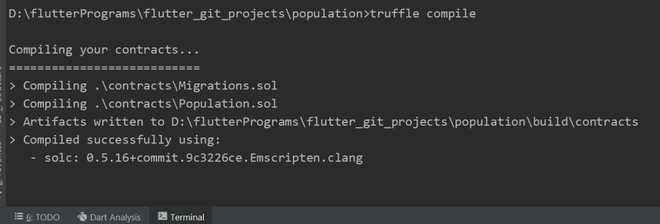truffle compile

### Migration

You’ll see one JavaScript file already in the migrations/ directory: 1_initial_migration.js. This handles deploying the Migrations.sol contract to observe subsequent smart contract migrations, and ensures we don’t double-migrate unchanged contracts in the future.

Let’s create our own migration script :

1. Create a new file named 2_deploy_contracts.js in the migrations/ directory.
2. Add the following content to the 2_deploy_contracts.js file:

## Javascript

 `const Population = artifacts.require(``"Population"``); ` ` `  `module.exports = ``function` `(deployer) { ` `  ``deployer.deploy(Population); ` `};`

• Before we can migrate our contract to the blockchain, we need to have a blockchain running. For this article, we’re going to use Ganache, a personal blockchain for Ethereum development you can use to deploy contracts, develop applications, and run tests. If you haven’t already, download Ganache and double-click the icon to launch the application. This will generate a blockchain running locally on port 7545.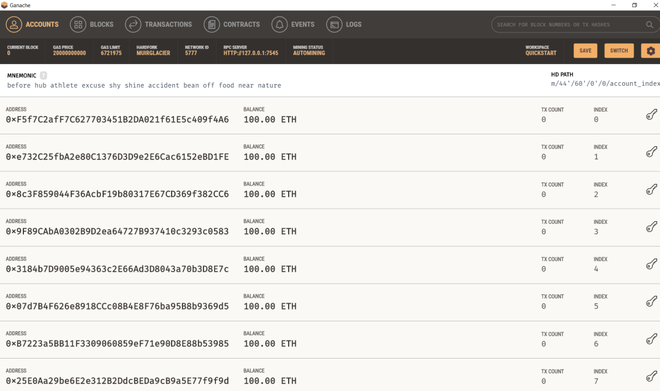Ganache

• Add the following content to the truffle-config.js file:

## Javascript

 `module.exports = {  ` `  ``networks: {  ` `     ``development: {  ` `      ``host: ``"127.0.0.1"``,     ``// Localhost (default: none)  ` `      ``port: 7545,            ``// Standard Ethereum port (default: none)  ` `      ``network_id: ``"*"``,       ``// Any network (default: none)  ` `     ``},  ` `  ``},  ` `    ``contracts_build_directory: ``"./src/artifacts/"``,  ` `       `  `  ``// Configure your compilers  ` `  ``compilers: {  ` `    ``solc: {      ` `       `  `       ``// See the solidity docs for advice  ` `       ``// about optimization and evmVersion  ` `        ``optimizer: {  ` `          ``enabled: ``false``,  ` `          ``runs: 200  ` `        ``},  ` `        ``evmVersion: ``"byzantium"` `    ``}  ` `  ``}  ` `};`

• Migrating the contract to the blockchain, run:
`truffle migrate`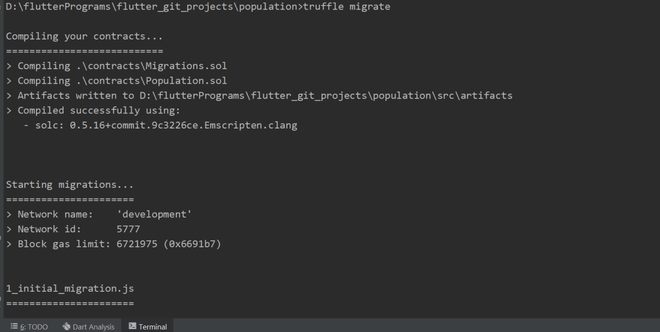truffle migrate

• Take a look into the Ganache, the first account originally had 100 ether, it is now lower, due to the transaction costs of migration.

## Testing the Smart Contract

In Truffle, we can write tests either in JavaScript or Solidity, In this article, we’ll be writing our tests in Javascript using the Chai and Mocha libraries.

1. Create a new file named population.js in the test/ directory.
2. Add the following content to the population.js file:

## Javascript

 `const Population = artifacts.require(``"Population"``) ; ` ` `  `contract(``"Population"` `, () =>{ ` `    ``let population = ``null` `; ` `    ``before(async () => { ` `        ``population = await Population.deployed() ; ` `    ``}); ` ` `  `    ``it(``"Setting Current Population"` `, async () => { ` `        ``await population.set(``"India"` `, 1388901219) ; ` `        ``const name = await population.countryName() ; ` `        ``const pop = await population.currentPopulation(); ` `        ``assert(name === ``"India"``) ; ` `        ``assert(pop.toNumber() === 1388901219) ; ` `    ``}); ` ` `  `    ``it(``"Decrement Current Population"` `, async () => { ` `        ``await population.decrement(100) ; ` `        ``const pop = await population.currentPopulation() ; ` `        ``assert(pop.toNumber() === 1388901119); ` `    ``}); ` ` `  `    ``it(``"Increment Current Population"` `, async () => { ` `            ``await population.increment(200) ; ` `            ``const pop = await population.currentPopulation() ; ` `            ``assert(pop.toNumber() === 1388901319); ` `        ``}); ` `}); `

• Population: The smart contract we want to test, We begin our test by importing our Population contract using artifacts.require.
• Testing the set,decrement and increment functions of Population.sol smart contract by providing it with some defined values.
• Truffle imports Chai so we can use the assert function. We pass the actual value and the expected value, To check that the name is set properly or not, assert(name === “India”) ; .
• For the currentPopulation, it returns BigNum object but Javascript doesn’t deal with it, Therefore converting the BigNum object to the regular Javascript object for check as assert(pop.toNumber() === 1388901219) ;

### Running the tests

• Running the test as:
`truffle test`
• If all the test pass, you’ll see the console output similar to this: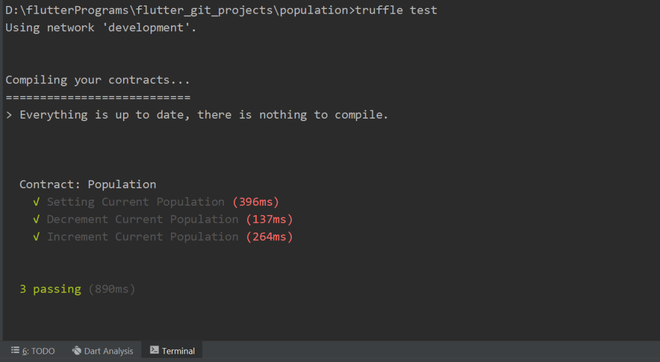truffle test

• In the pubspec.yaml file import the following packages :
```provider: ^4.3.3
web3dart: ^1.2.3
http: ^0.12.2
web_socket_channel: ^1.2.0
country_pickers: ^1.3.0```
• Also, add the asset src/artifacts/Population.json to pubspec.yaml file which is generated by truffle-config.js while we migrate our contract.
```assets:
- src/artifacts/Population.json```
1. Create a new file named contract_linking.dart in the lib/ directory.
2. Add the following content to the file:

## Dart

 `import ``'package:flutter/foundation.dart'``; ` ` `  `class` `ContractLinking extends ChangeNotifier { ` `   `  `}`

### Variables

• Add the following variable on the next line after class ContractLinking extends ChangeNotifier {

## Dart

 `final String _rpcUrl = ``"http://10.0.2.2:7545"``; ` `final String _wsUrl = ``"ws://10.0.2.2:7545/"``; ` `final String _privateKey = ``"Enter Private Key"``;`

The library web3dart won’t send signed transactions to miners itself. Instead, it relies on an RPC client to do that.For the WebSocket url just modify the RPC url. You can get the RPC url from the ganache :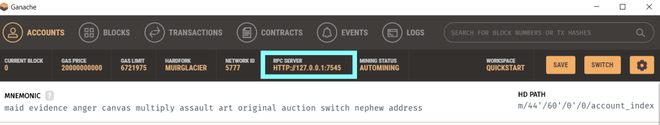Ganache – RPC url

• Get the Private Key from ganache: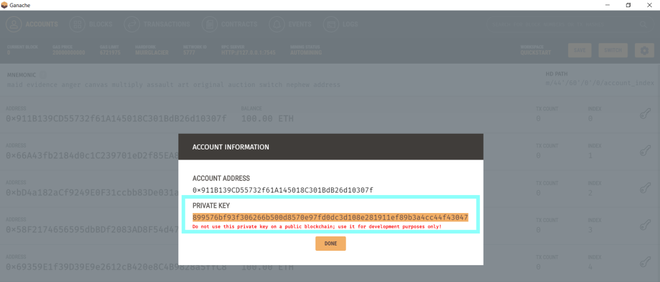Ganache – Private Key

• Declare the following variable’s below :

## Dart

 `Web3Client _client; ` `bool` `isLoading = ``true``; `   `String _abiCode; ` `EthereumAddress _contractAddress; `   `Credentials _credentials; `   `DeployedContract _contract; ` `ContractFunction _countryName; ` `ContractFunction _currentPopulation; ` `ContractFunction _set; ` `ContractFunction _decrement; ` `ContractFunction _increment; `   `String countryName; ` `String currentPopulation;`

1. _client variable will be used to establish a connection to the ethereum rpc node with the help of WebSocket.
2. isLoading variable will be used to check the state of the contract.
3. _abiCode variable will be used to, read the contract abi.
4. _contractAddress variable will be used to store the, contract address of the deployed smart contract.
5. _credentials variable will store the, credentials of the smart contract deployer.
6. _contract variable will be used to tell Web3dart where our smart contract is declared.
7. _countryName , _currentPopulation , _set , _decrement , and _increment variables will be used to hold the functions declared in our Population.sol smart contract.
8. countryName and currentPopulation will hold the exact name and population from the smart contract.

### Functions

• After declaring the above variable’s, Declare the following functions below it :

## Dart

 `ContractLinking() { ` `  ``initialSetup(); ` `} `   `initialSetup() async { ` `   `  `  ``// establish a connection to the ethereum rpc node. The socketConnector ` `  ``// property allows more efficient event streams over websocket instead of ` `  ``// http-polls. However, the socketConnector property is experimental. ` `  ``_client = Web3Client(_rpcUrl, Client(), socketConnector: () { ` `    ``return` `IOWebSocketChannel.connect(_wsUrl).cast(); ` `  ``}); ` `  ``await getAbi(); ` `  ``await getCredentials(); ` `  ``await getDeployedContract(); ` `} `   `Future<``void``> getAbi() async { ` `   `  `  ``// Reading the contract abi ` `  ``final abiStringFile = ` `      ``await rootBundle.loadString(``"src/artifacts/Population.json"``); ` `  ``final jsonAbi = jsonDecode(abiStringFile); ` `  ``_abiCode = jsonEncode(jsonAbi[``"abi"``]); ` `  ``_contractAddress = ` `      ``EthereumAddress.fromHex(jsonAbi[``"networks"``][``"5777"``][``"address"``]); ` `} `   `Future<``void``> getCredentials() async { ` `  ``_credentials = await _client.credentialsFromPrivateKey(_privateKey); ` `} `   `Future<``void``> getDeployedContract() async { ` `   `  `  ``// Telling Web3dart where our contract is declared. ` `  ``_contract = DeployedContract( ` `      ``ContractAbi.fromJson(_abiCode, ``"Population"``), _contractAddress); ` `   `  `  ``// Extracting the functions, declared in contract. ` `  ``_countryName = _contract.function(``"countryName"``); ` `  ``_currentPopulation = _contract.function(``"currentPopulation"``); ` `  ``_set = _contract.function(``"set"``); ` `  ``_decrement = _contract.function(``"decrement"``); ` `  ``_increment = _contract.function(``"increment"``); `   `  ``getData(); ` `} `   `getData() async { ` `   `  `  ``// Getting the current name and population declared in the smart contract. ` `  ``List name = await _client ` `      ``.call(contract: _contract, function: _countryName, params: []); ` `  ``List population = await _client ` `      ``.call(contract: _contract, function: _currentPopulation, params: []); ` `  ``countryName = name; ` `  ``currentPopulation = population.toString(); ` `  ``print(``"\$countryName , \$currentPopulation"``); ` `  ``isLoading = ``false``; ` `  ``notifyListeners(); ` `} `   `addData(String nameData, BigInt countData) async { ` `   `  `  ``// Setting the countryName  and currentPopulation defined by the user ` `  ``isLoading = ``true``; ` `  ``notifyListeners(); ` `  ``await _client.sendTransaction( ` `      ``_credentials, ` `      ``Transaction.callContract( ` `          ``contract: _contract, ` `          ``function: _set, ` `          ``parameters: [nameData, countData])); ` `  ``getData(); ` `} `   `increasePopulation(``int` `incrementBy) async { ` `   `  `  ``// Increasing the currentPopulation ` `  ``isLoading = ``true``; ` `  ``notifyListeners(); ` `  ``await _client.sendTransaction( ` `      ``_credentials, ` `      ``Transaction.callContract( ` `          ``contract: _contract, ` `          ``function: _increment, ` `          ``parameters: [BigInt.from(incrementBy)])); ` `  ``getData(); ` `} `   `decreasePopulation(``int` `decrementBy) async { ` `   `  `  ``// Decreasing the currentPopulation ` `  ``isLoading = ``true``; ` `  ``notifyListeners(); ` `  ``await _client.sendTransaction( ` `      ``_credentials, ` `      ``Transaction.callContract( ` `          ``contract: _contract, ` `          ``function: _decrement, ` `          ``parameters: [BigInt.from(decrementBy)])); ` `  ``getData(); ` `}`

## Creating a UI to interact with the smart contract

1. Create a new file named display_population.dart in the lib/ directory.
2. Add the following content to the file:

## Dart

 `import ``'package:country_pickers/utils/utils.dart'``; ` `import ``'package:flutter/material.dart'``; ` `import ``'package:population/set_population.dart'``; ` `import ``'package:provider/provider.dart'``; ` `import ``'package:population/contract_linking.dart'``; ` ` `  `class` `DisplayPopulation extends StatelessWidget { ` `  ``@override ` `  ``Widget build(BuildContext context) { ` `    ``final contractLink = Provider.of(context); ` ` `  `    ``return` `Scaffold( ` `      ``appBar: AppBar( ` `        ``title: Text(``"Population On Blockchain"``), ` `        ``centerTitle: ``true``, ` `      ``), ` `      ``floatingActionButton: FloatingActionButton( ` `        ``child: Icon(Icons.edit), ` `        ``onPressed: () { ` `          ``Navigator.push( ` `              ``context, ` `              ``MaterialPageRoute( ` `                  ``builder: (context) => SetPopulation(), ` `                  ``fullscreenDialog: ``true``)); ` `        ``}, ` `      ``), ` `      ``body: Container( ` `        ``padding: EdgeInsets.symmetric(horizontal: 20), ` `        ``child: Center( ` `          ``child: contractLink.isLoading ` `              ``? CircularProgressIndicator() ` `              ``: SingleChildScrollView( ` `                  ``child: Column( ` `                    ``children: [ ` `                      ``contractLink.countryName == ``"Unknown"` `                          ``? Icon( ` `                              ``Icons.error, ` `                              ``size: 100, ` `                            ``) ` `                          ``: Container( ` `                              ``child: CountryPickerUtils.getDefaultFlagImage( ` `                                  ``CountryPickerUtils.getCountryByIsoCode( ` `                                      ``contractLink.countryName)), ` `                              ``width: 250, ` `                              ``height: 150, ` `                            ``), ` `                      ``Padding( ` `                        ``padding: ``const` `EdgeInsets.all(8.0), ` `                        ``child: Text( ` `                          ``"Country - \${contractLink.countryName}"``, ` `                          ``style: TextStyle( ` `                              ``fontSize: 30, ` `                              ``fontWeight: FontWeight.bold, ` `                              ``color: Theme.of(context).accentColor), ` `                        ``), ` `                      ``), ` `                      ``Padding( ` `                        ``padding: ``const` `EdgeInsets.all(8.0), ` `                        ``child: Text( ` `                            ``"Population - \${contractLink.currentPopulation}"``, ` `                            ``style: TextStyle( ` `                                ``fontSize: 25, ` `                                ``fontWeight: FontWeight.bold, ` `                                ``color: Theme.of(context).primaryColor)), ` `                      ``), ` `                      ``contractLink.countryName == ``"Unknown"` `                          ``? Text(``""``) ` `                          ``: Padding( ` `                              ``padding: ``const` `EdgeInsets.all(8.0), ` `                              ``child: Row( ` `                                ``mainAxisAlignment: MainAxisAlignment.center, ` `                                ``children: [ ` `                                  ``ElevatedButton.icon( ` `                                    ``onPressed: () { ` `                                      ``dialog(context, ``"Increase"``); ` `                                    ``}, ` `                                    ``icon: ` `                                        ``Icon(Icons.person_add_alt_1, size: 18), ` `                                    ``label: Text(``"Increase"``), ` `                                  ``), ` `                                  ``SizedBox( ` `                                    ``width: 15, ` `                                  ``), ` `                                  ``ElevatedButton.icon( ` `                                    ``onPressed: () { ` `                                      ``if` `(contractLink.currentPopulation != ` `                                          ``"0"``) { ` `                                        ``dialog(context, ``"Decrease"``); ` `                                      ``} ` `                                    ``}, ` `                                    ``icon: Icon(Icons.person_remove_alt_1, ` `                                        ``size: 18), ` `                                    ``label: Text(``"Decrease"``), ` `                                  ``) ` `                                ``], ` `                              ``), ` `                            ``) ` `                    ``], ` `                  ``), ` `                ``), ` `        ``), ` `      ``), ` `    ``); ` `  ``} ` ` `  `  ``dialog(context, method) { ` `    ``final contractLink = Provider.of(context, listen: ``false``); ` `    ``TextEditingController countController = TextEditingController(); ` `    ``showDialog( ` `        ``context: context, ` `        ``builder: (_) => AlertDialog( ` `              ``title: method == ``"Increase"` `                  ``? Text(``"Increase Population"``) ` `                  ``: Text(``"Decrease Population"``), ` `              ``content: Column( ` `                ``mainAxisSize: MainAxisSize.min, ` `                ``crossAxisAlignment: CrossAxisAlignment.start, ` `                ``children: [ ` `                  ``Text( ` `                      ``"Current Population is \${contractLink.currentPopulation}"``), ` `                  ``Padding( ` `                    ``padding: EdgeInsets.only(top: 20.0), ` `                    ``child: TextField( ` `                      ``controller: countController, ` `                      ``keyboardType: TextInputType.number, ` `                      ``decoration: InputDecoration( ` `                        ``border: OutlineInputBorder(), ` `                        ``hintText: method == ``"Increase"` `                            ``? ``"Increase Population By ..."` `                            ``: ``"Decrease Population By ..."``, ` `                      ``), ` `                    ``), ` `                  ``) ` `                ``], ` `              ``), ` `              ``actions: [ ` `                ``Row( ` `                  ``children: [ ` `                    ``TextButton( ` `                      ``child: Text(``"Cancel"``), ` `                      ``onPressed: () { ` `                        ``Navigator.of(context).pop(); ` `                      ``}, ` `                    ``), ` `                    ``TextButton( ` `                      ``child: method == ``"Increase"` `                          ``? Text(``"Increase"``) ` `                          ``: Text(``"Decrease"``), ` `                      ``onPressed: () { ` `                        ``method == ``"Increase"` `                            ``? contractLink.increasePopulation( ` `                                ``int``.parse(countController.text)) ` `                            ``: contractLink.decreasePopulation( ` `                                ``int``.parse(countController.text)); ` `                        ``Navigator.of(context).pop(); ` `                      ``}, ` `                    ``), ` `                  ``], ` `                ``) ` `              ``], ` `            ``)); ` `  ``} ` `} `

1. Create another new file named set_population.dart in the lib/ directory.
2. Add the following content to the file:

## Dart

 `import ``'package:country_pickers/country.dart'``; ` `import ``'package:country_pickers/country_pickers.dart'``; ` `import ``'package:flutter/material.dart'``; ` `import ``'package:population/contract_linking.dart'``; ` `import ``'package:provider/provider.dart'``; ` ` `  `class` `SetPopulation extends StatefulWidget { ` `  ``@override ` `  ``_SetPopulationState createState() => _SetPopulationState(); ` `} ` ` `  `class` `_SetPopulationState extends State { ` `  ``Country _selectedCountry = CountryPickerUtils.getCountryByIsoCode(``'AF'``); ` ` `  `  ``TextEditingController countryNameController = ` `      ``TextEditingController(text: ``"Unknown"``); ` `  ``TextEditingController populationController = TextEditingController(); ` ` `  `  ``@override ` `  ``Widget build(BuildContext context) { ` `    ``final contractLink = Provider.of(context); ` ` `  `    ``return` `Scaffold( ` `        ``appBar: AppBar( ` `          ``title: Text(``"Set Population"``), ` `          ``actions: [ ` `            ``TextButton( ` `                ``onPressed: () { ` `                  ``contractLink.addData(countryNameController.text, ` `                      ``BigInt.from(``int``.parse(populationController.text))); ` `                  ``Navigator.pop(context); ` `                ``}, ` `                ``child: Text( ` `                  ``"SAVE"``, ` `                  ``style: TextStyle( ` `                      ``color: Colors.brown, fontWeight: FontWeight.bold), ` `                ``)) ` `          ``], ` `        ``), ` `        ``body: Container( ` `          ``child: Center( ` `            ``child: SingleChildScrollView( ` `              ``child: Column( ` `                ``children: [ ` `                  ``stackCard(countryFlagPicker(), countryFlag()), ` `                  ``stackCard(populationTextfield(), countryFlag()), ` `                ``], ` `              ``), ` `            ``), ` `          ``), ` `        ``)); ` `  ``} ` ` `  `  ``Widget stackCard(Widget widget1, Widget widget2) { ` `    ``return` `Stack( ` `      ``children: [ ` `        ``Padding( ` `          ``padding: EdgeInsets.only(bottom: 8.0, left: 54), ` `          ``child: Container( ` `            ``height: 120, ` `            ``child: Card( ` `              ``color: Colors.cyan, ` `              ``child: Column( ` `                ``crossAxisAlignment: CrossAxisAlignment.start, ` `                ``mainAxisAlignment: MainAxisAlignment.spaceAround, ` `                ``children: [widget1], ` `              ``), ` `            ``), ` `          ``), ` `        ``), ` `        ``widget2 ` `      ``], ` `    ``); ` `  ``} ` ` `  `  ``Widget countryFlag() { ` `    ``return` `Positioned( ` `      ``top: 15, ` `      ``child: Container( ` `        ``width: 100, ` `        ``height: 100, ` `        ``decoration: BoxDecoration( ` `          ``shape: BoxShape.circle, ` `        ``), ` `        ``child: CircleAvatar( ` `            ``backgroundColor: Colors.blueGrey, ` `            ``child: Container( ` `                ``width: 80, ` `                ``height: 50, ` `                ``child: ` `                    ``CountryPickerUtils.getDefaultFlagImage(_selectedCountry))), ` `      ``), ` `    ``); ` `  ``} ` ` `  `  ``Widget countryFlagPicker() { ` `    ``return` `CountryPickerDropdown( ` `      ``underline: Container( ` `        ``height: 2, ` `        ``color: Colors.black, ` `      ``), ` `      ``onValuePicked: (Country country) { ` `        ``print(``"\${country.name}"``); ` `        ``setState(() { ` `          ``_selectedCountry = country; ` `          ``countryNameController.text = country.isoCode; ` `        ``}); ` `      ``}, ` `      ``itemBuilder: (Country country) { ` `        ``return` `Row( ` `          ``children: [ ` `            ``SizedBox(width: 48.0), ` `            ``CountryPickerUtils.getDefaultFlagImage(country), ` `            ``SizedBox(width: 8.0), ` `            ``Expanded( ` `                ``child: Text( ` `              ``country.name, ` `              ``style: TextStyle( ` `                  ``color: Colors.brown, ` `                  ``fontSize: 25, ` `                  ``fontWeight: FontWeight.bold), ` `            ``)), ` `          ``], ` `        ``); ` `      ``}, ` `      ``icon: Icon( ` `        ``Icons.arrow_downward, ` `        ``color: Colors.white, ` `        ``size: 50, ` `      ``), ` `      ``itemHeight: null, ` `      ``isExpanded: ``true``, ` `    ``); ` `  ``} ` ` `  `  ``Widget populationTextfield() { ` `    ``return` `Padding( ` `      ``padding: EdgeInsets.only(left: 48.0, right: 5), ` `      ``child: TextField( ` `        ``decoration: InputDecoration( ` `            ``filled: ``true``, ` `            ``fillColor: Colors.black, ` `            ``focusedBorder: OutlineInputBorder(), ` `            ``labelText: ``"Population"``, ` `            ``labelStyle: TextStyle(color: Colors.white), ` `            ``hintText: ``"Enter Population"``, ` `            ``prefixIcon: Icon(Icons.person_pin_outlined)), ` `        ``keyboardType: TextInputType.number, ` `        ``controller: populationController, ` `      ``), ` `    ``); ` `  ``} ` `} `

• Update the main.dart as :

## Dart

 `import ``'package:flutter/cupertino.dart'``; ` `import ``'package:flutter/material.dart'``; ` `import ``'package:population/display_population.dart'``; ` `import ``'package:provider/provider.dart'``; ` `import ``'package:population/contract_linking.dart'``; ` ` `  `void` `main() => runApp(MyApp()); ` ` `  `class` `MyApp extends StatelessWidget { ` `  ``@override ` `  ``Widget build(BuildContext context) { ` `    ``return` `ChangeNotifierProvider( ` `      ``create: (_) => ContractLinking(), ` `      ``child: MaterialApp( ` `        ``title: ``'Population On Blockchain'``, ` `        ``theme: ThemeData( ` `            ``brightness: Brightness.dark, ` `            ``primaryColor: Colors.cyan, ` `            ``accentColor: Colors.deepOrange), ` `        ``home: DisplayPopulation(), ` `      ``), ` `    ``); ` `  ``} ` `}`

## Interacting with the complete Dapp

1. Now we’re ready to use our dapp!
2. Just RUN the Flutter Project.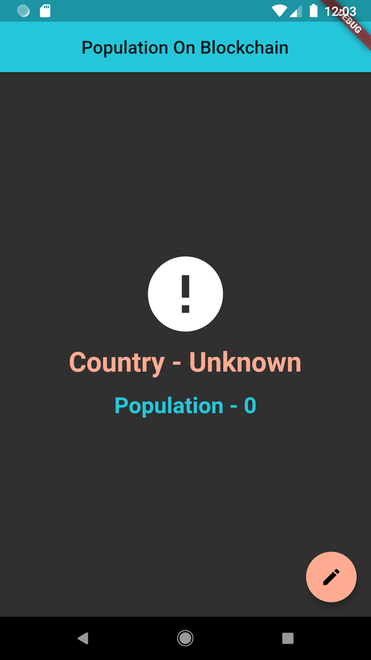As you can see the countryName (Unknown) and currentPopulation (0) is actually coming from the smart-contract, which we’d set in the constructor of our smart contract.

When you click on the floatingActionButton , it will push the current route to SetPopulation().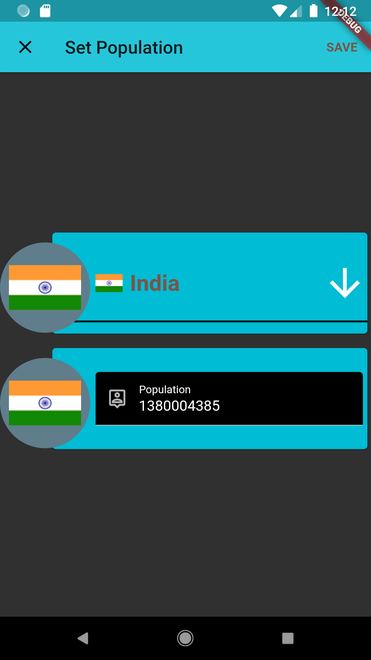1. You can define the countryName and currentPopulation here.
2. When you click on save, the specified name and population will be actually added to the blockchain.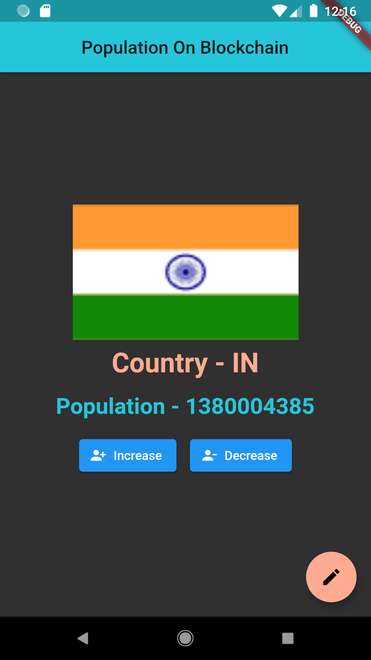• You can Increase or Decrease the currentPopulation by just click on the ElevatedButton as specified.

Congratulations! You have taken a huge step to become a full-fledged mobile dapp developer. For developing locally, you have all the tools you need to start making more advanced dapps.

If you stuck somewhere, do check out the GitHub repository for complete code.

My Personal Notes arrow_drop_up
Related Articles